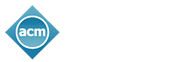#### A PHP Error was encountered

Severity: 8192

Message: Non-static method URL_tube::usage() should not be called statically, assuming \$this from incompatible context

Filename: url_tube/pi.url_tube.php

Line Number: 13

KDD 2020 | Measuring Model Complexity of Neural Networks with Curve Activation Functions

## Measuring Model Complexity of Neural Networks with Curve Activation Functions

Xia Hu: Simon Fraser University and Microsoft Research Asia; Weiqing Liu: Microsoft Research Asia; Jiang Bian: Microsoft Research Asia; Jian Pei: Simon Fraser University

It is fundamental to measure model complexity of deep neural networks. A good model complexity measure can help to tackle many challenging problems, such as overfitting detection, model selection, and performance improvement. The existing literature on model complexity mainly focuses on neural networks with piecewise linear activation functions. Model complexity of neural networks with general curve activation functions remains an open problem. To tackle the challenge, in this paper, we first propose linear approximation neural network (LANN for short), a piecewise linear framework to approximate a given deep model with curve activation function. LANN constructs individual piecewise linear approximation for the activation function of each neuron, and minimizes the number of linear regions to satisfy a required approximation degree. Then, we analyze the upper bound of the number of linear regions formed by LANNs, and derive the complexity measure based on the upper bound. To examine the usefulness of the complexity measure, we experimentally explore the training process of neural networks and detect overfitting. Our results demonstrate that the occurrence of overfitting is positively correlated with the increase of model complexity during training. We find that the L1 and L2 regularizations suppress the increase of model complexity. Finally, we propose two approaches to prevent overfitting by directly constraining model complexity, namely neuron pruning and customized L1 regularization.

## How can we assist you?

We'll be updating the website as information becomes available. If you have a question that requires immediate attention, please feel free to contact us. Thank you!

Please enter the word you see in the image below: